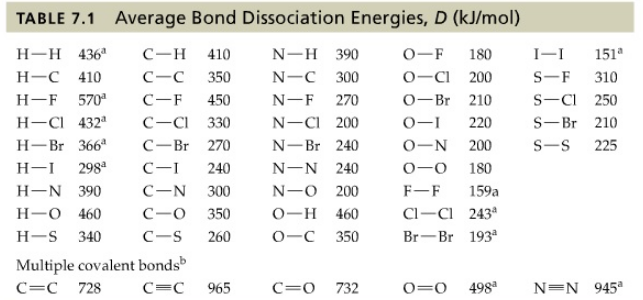# Problem: Use the bond dissociation energies Table 7.1 to calculate an approximate ΔH o (in kilojoules) for the reaction of ethylene with hydrogen to yield ethane.H2C=CH2(g)+H2(g) → CH3CH3(g)

###### FREE Expert Solution

We're asked to calculate an approximate ΔHo (in kilojoules) for the reaction of ethylene with hydrogen to yield ethane:

H2C=CH2(g) + H2(g) → CH3CH3(g)

Recall that we can solve for the ΔH°rxn using bond energies, using the equation:

$\overline{){\mathbf{∆}}{\mathbf{H}}{{\mathbf{°}}}_{{\mathbf{rxn}}}{\mathbf{=}}{{\mathbf{H}}}_{\mathbf{bond}\mathbf{,}\mathbf{reactants}}{\mathbf{-}}{{\mathbf{H}}}_{\mathbf{bond}\mathbf{,}\mathbf{products}}}$

To do that, we need to do these steps:

Step 1: Draw the Lewis structure of each compound and determine the type of bonds present

Step 2: Solve for ΔH°rxn using the given bond energies

91% (320 ratings)###### Problem Details

Use the bond dissociation energies Table 7.1 to calculate an approximate ΔH o (in kilojoules) for the reaction of ethylene with hydrogen to yield ethane.

H2C=CH2(g)+H2(g) → CH3CH3(g)